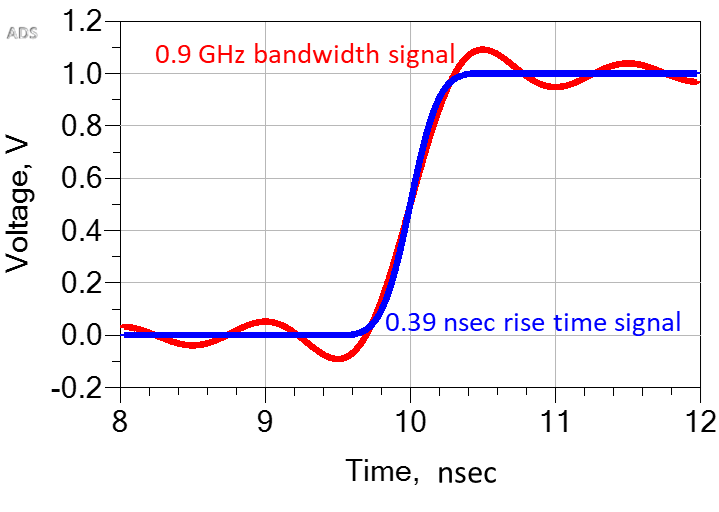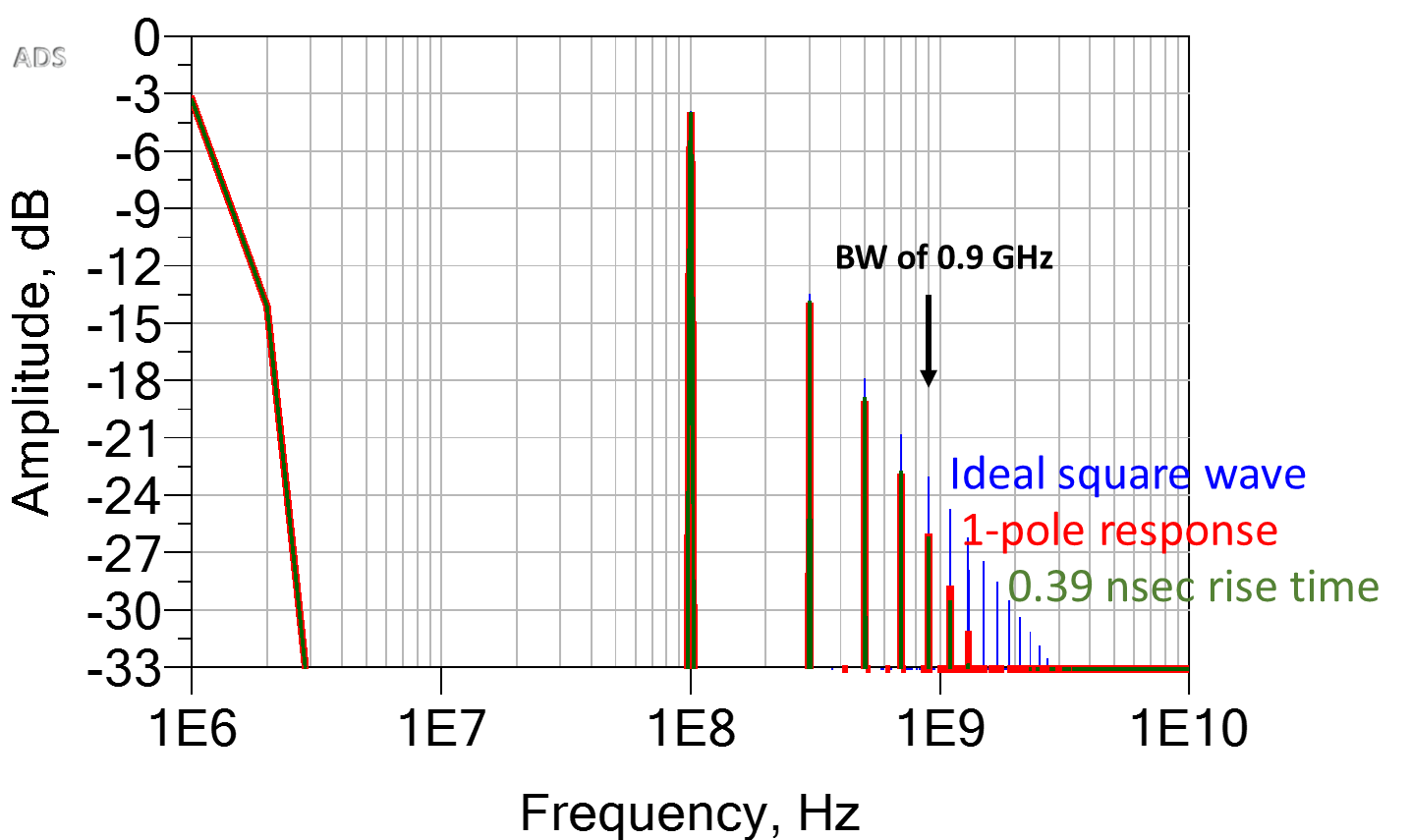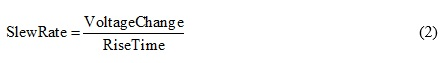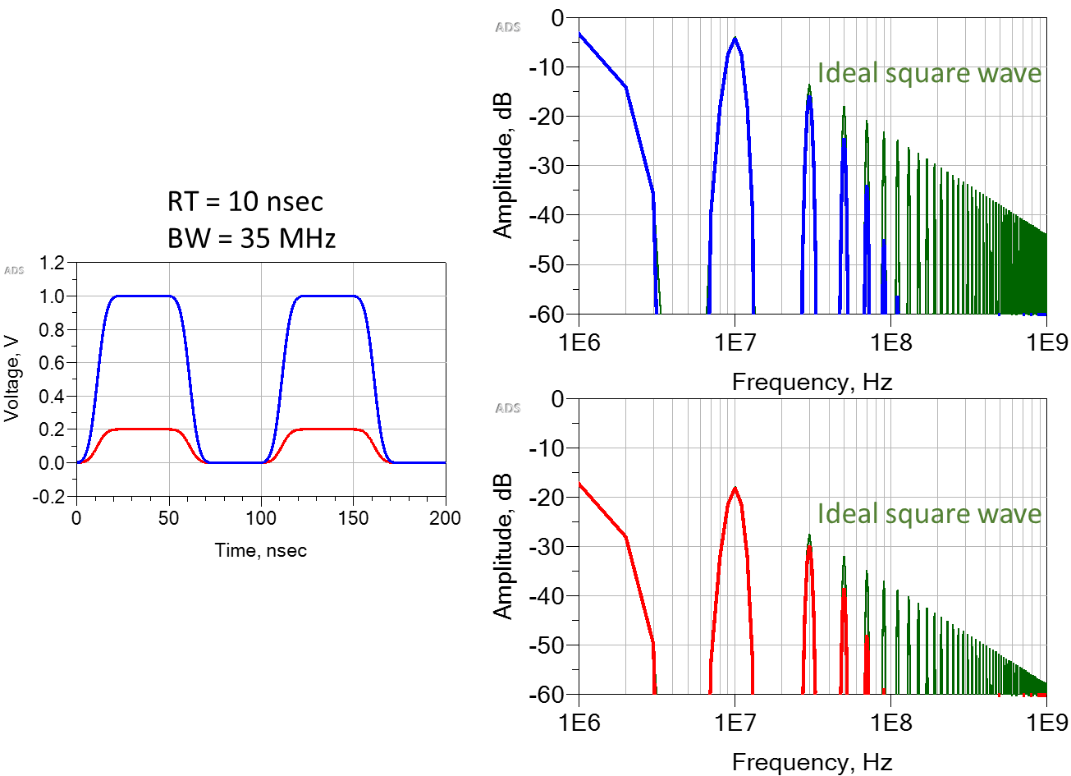The quality of the 1-pole model depends on the precise shape of the time domain waveform and the definition of bandwidth. For the specific case of the synthesized square wave with a 100 MHz repeat frequency, the bandwidth of the synthesized signal with just the first 9 harmonics added together is 0.9 GHz. The measured 10 to 90 percent rise time is 0.39 nsec. A signal with the specific shape of an error function rising edge (the integral of a Gaussian) with a 10 to 90 percent rise time is also 0.39 nsec (see Figure 7). The signals are not exactly the same shape but are similar and should have a similar bandwidth.Fig. 7 Comparison of a 0.39 nsec rise time waveform with a finite rise time square wave including frequency components up to 0.9 GHz.

The spectrum of the error function signal with a rise time of 0.39 nsec also looks like the spectrum of an ideal square wave through a 1-pole filter (see Figure 8). The amplitude of the frequency component at the signal’s bandwidth, 0.9 GHz, is also down 3 dB from the ideal square wave; and, above 0.9 GHz, the frequency components of the error function edge signal drop off faster than 20 dB/decade. It is as though the amplitudes are insignificant above the pole frequency, like in the synthesized waveform.Fig. 8 Spectrum of an ideal square wave, dropping off as 1/f, the 1-pole response of the ideal square wave, and the spectrum of a 0.39 nsec rise time signal. The scale is 3 dB/div.

The similarity in the spectral features of : 1) an ideal square wave through a 1-pole filter with a 10 to 90 percent rise time step response, 2) the spectrum of an error function edge signal with a 0.39 nsec rise time, and 3) the spectrum of a synthesized square wave with a 0.39 nsec rise time

illustrates that the pole frequency of a filter is a useful approximation for determining the highest frequency component needed to recreate a waveform in the time domain. It is, however, just an approximation.

This is the origin of the rule of thumb, for example, that the bandwidth of a 1 nsec rise time signal is 0.35/1 nsec = 350 MHz. Given this approximation for bandwidth, what is the slew rate?

### Rise time and slew rate

The slew rate of a signal is the slope of the voltage waveform, i.e., the rate of change of the voltage. To first order, slew rate is:A signal with a slew rate independent of the rise time can be created by scaling the voltage change in magnitude. For example, in Figure 1 two waveforms are shown. They have the same 10 to 90 percent rise times of 10 nsec, but the amplitude of the larger one is 5x the smaller one. The larger waveform, therefore, has a slew rate 5x that of the smaller one.

The larger amplitude signal has a much larger dV/dt. It looks like it should have higher frequency components in its spectrum to generate the larger slope, but the rise times are equal. When the amplitudes of the waveforms are scaled, the amplitudes of the spectral components are scaled as well. The frequency at which the amplitude drops by 3 dB for the corresponding ideal square waves is the same (see Figure 9).Fig. 9 The spectrums of the two waveforms compared to their corresponding ideal square waves. The frequency at which the amplitudes are down by 3 dB are identical; therefore, the bandwidths of the two waveforms are identical.

The bandwidth of each waveform is related to the shape of the spectrum, not the absolute magnitude of the frequency components. Recreating the finite rise time square wave with a larger amplitude requires the same frequency components as the small amplitude signal, each component just has a larger amplitude. The highest frequency component needed to recreate each waveform is the same.

### Conclusion

Every signal in the time domain has its own corresponding spectrum in the frequency domain. In the special case of an ideal square wave, the spectral components and their amplitudes are easily calculated. To create an ideal square wave with a 0 psec rise time requires an infinite number of sinusoidal frequency components. Even though the amplitude of these components becomes smaller and smaller with increasing frequency, each one is needed to create a short rise time. The absolute magnitude of a frequency component is not what determines its bandwidth.

In a synthesized, finite rise time square wave, no frequency components are required above the bandwidth to recreate the rise time. Empirically, this bandwidth is found to be close to the commonly used model for the bandwidth of a signal based on the step response of a 1-pole filter.

The spectrum of a signal depends on the precise shape of the waveform. In practice this spectrum is approximated by the step response of a 1-pole filter. The pole frequency is the frequency where the amplitude drops by 3 dB compared to an ideal square wave. The contributions of higher frequency components thereafter are negligible.

Since it is the rise time of a signal that influences bandwidth, the slope of the voltage change is not a factor. If the amplitude signal of a signal doubles, the slew rate doubles, but the amplitude of every harmonic component also doubles. The frequency components 3 dB down from the equivalent ideal square wave, and thereafter drop more rapidly, are the same.

### References

1. E. Bogatin, “Back to Basics: Bandwidth and Rise Time,” Signal Integrity Journal, May 2018. Web.